# How to convert date to text in Excel with TEXT function and without formulas

In the previous article, we discussed different ways to convert text to date in Excel. If you are looking for a solution to the opposite task - changing an Excel date to text - a few choices are available to you again.

Traditionally, we'll begin with a formula solution and then explore a couple of non-formula ways.

## Using TEXT function in Excel to convert date to text

The Excel TEXT function is specially designed to convert a numeric value to a text string and display it in the format you specify.

The syntax of the Excel TEXT function is as follows:

TEXT(value, format_text)

Where:

• `value` is a numeric value you want to convert to text. This can be a number, a formula that returns a numeric value, or a reference to a cell containing a number.
• `format_text` this is how you want to format the resulting text value, provided as a text string enclosed in quotation marks.

For example, you can use the following formula to convert a date in cell A1 to a text string in the traditional US date format (month/day/year):

`=TEXT(A1,"mm/dd/yyyy")`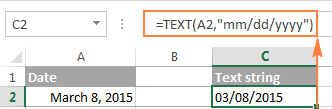As you see in the screenshot above, the value returned by the TEXT formula is aligned to the left, which is the first sign that points to a date formatted as text. Apart from alignment in a cell, there are a few more indicators that can help you distinguish between dates and text strings in Excel.

### Example 1. How to convert date to text strings in different formats

Since Excel dates are serial numbers in their nature, the Excel TEXT function has no problem with converting them to text values. The most challenging part is probably specifying the proper display formatting for the text dates.

Microsoft Excel understands the following date codes.

Months:

• m - month number without a leading zero
• mm - month number with a leading zero
• mmm - short form of the month name, for example Mar
• mmmm - full form of the month name, for example March
• mmmmm - month as the first letter, for example M (stands for March and May)

Days:

• d - days number without a leading zero
• dd - day number with a leading zero
• ddd - abbreviated day of the week, for example Sun
• dddd - full name of the day of the week, for example Sunday

Years:

• yy - two-digit year
• yyyy - four-digit year

To display the converted text date exactly the way you want, you can separate the date codes with various delimiters such as dash (-), slash (/), comma (,) colon (:), etc. Here are a few examples:

• "mm/dd/yyyy" - the date format used in the USA, displays as 03/08/2015
• "dd/mm/yyyy" - the date format used by the rest of the world, displays as 08/03/2015
• "dd-mmm-yy" - displays as 08-Mar-15 to avoid any confusion : )
• "dddd, mmmm d, yyyy" - full date, including the day of the week, displays as Sunday, March 08, 2015

For example, if you have a column of US dates in Excel and you need to export them to a .csv file for your UK based partner, you can convert the dates to the UK format, as a courtesy:

`=TEXT(A1,"mm/dd/yyyy")`

Some more formula examples and their results are shown below: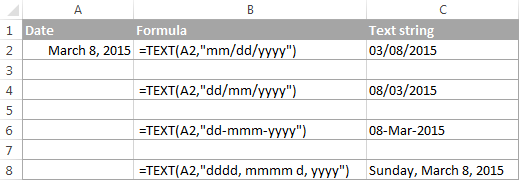### Example 2. How to convert time to text strings

If your date entries display both dates and times and you want to change them to text strings exactly as they are, you included the following time codes in the format_text argument of the Excel TEXT function.

Hours:

• h - hours without a leading zero, as 0-23.
• hh - hours with a leading zero, as 00-23.

Minutes:

• m - minutes without a leading zero, as 0-59
• mm - minutes with a leading zero, as 00-59

Seconds:

• s - seconds without a leading zero
• ss - seconds with a leading zero

Periods of the day:

• AM/PM - displays as AM or PM
• If not specified, 24-hour time format is used

As you probably noticed, the m codes are used for months as well as minutes, and you might be curious how Microsoft Excel distinguishes between them. If you put "m" immediately after h codes (hours) or immediately before s codes (seconds), Excel understands you want to display minutes rather than a month. Yep, it's that simple : )

The TEXT function in Excel allows including both date and time codes in the `format_text` argument, for example:

`=TEXT(A2,"dd/mm/yyyy hh:mm")`

If you want to convert the time portion only, then put only the time codes, like this:

`=TEXT(A2,"h:mm AM/PM")`

The results of your TEXT formulas may look similar to this: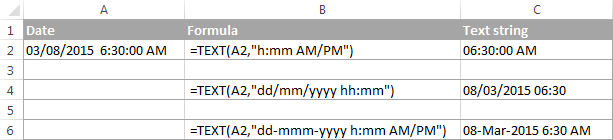### Example 3. How to convert the current date to text in Excel

In case you want to convert the current date to the text format, you can use the Excel TEXT function in combination with the TODAY function that returns the current date, for example:

`=TEXT(TODAY(), "dd-mmm-yyyy")`

The result of this formula would show up as 08-Mar-2015. If you prefer to display the resulting text string in some other format, please see the date codes discussed in Example 1.

### Example 4. Excel TEXT formula to convert text to date

Though the main destination of the TEXT function in Excel is converting numbers to text, it can also perform a reverse conversion, i.e. change text to date. For this, you simply add the double negation (--) to your TEXT formula.

For example, to convert a text string in cell A1 to date, you use the below formula, and then format the cell as a date.

`=--TEXT(A1,"mm/dd/yy")`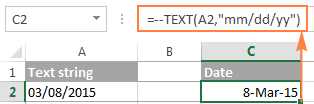Note. In the above examples, we used the date and time codes for the English locale of Excel. If you have a different locale, the codes may be different for your language.

## Converting date to text with Excel's Text to Columns wizard

As you've just seen, Excel's TEXT function makes a good job of converting dates to text. But if you are not a big fan of Excel formulas, you might like this solution better.

If you had a chance to read the previous part of our Excel dates tutorial, you already know how to use Text to Columns to change text to date. To convert dates to text strings, you proceed in the same way with the only difference that you choose Text instead of Date on the final step of the wizard.

Note. The Text to Column wizard always converts dates in the default short date format regardless of how the original dates are displayed in your worksheet. You can find more about default date and time formats in the following article: Default date format in Excel.

If the default date format is not what you are looking for, you can jump right to the next solution that lets you convert dates to text strings in any format of your choosing.

If you don't mind the default format, then perform the following steps:

1. In your Excel spreadsheet, select all of the dates you want to change to text.
2. On the Data tab, find the Data Tools group, and click Text to Columns.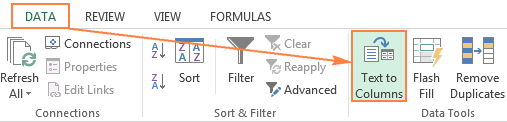3. On step 1 of the wizard, select the Delimited file type and click Next.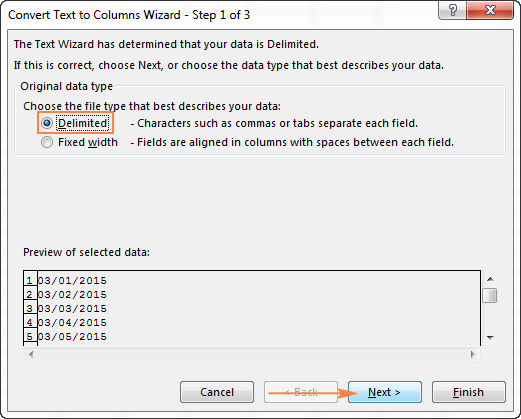4. On step 2 of the wizard, make sure none of the delimiter boxes is checked and click Next.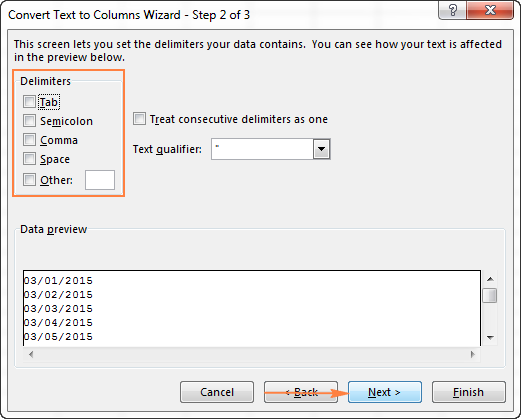5. On step 3 of the wizard, which is the final step, select Text under Column data format and click Finish.

Tip. If you don't want the resulting text strings to overwrite the original dates, specify the Destination for the top cell of the new column.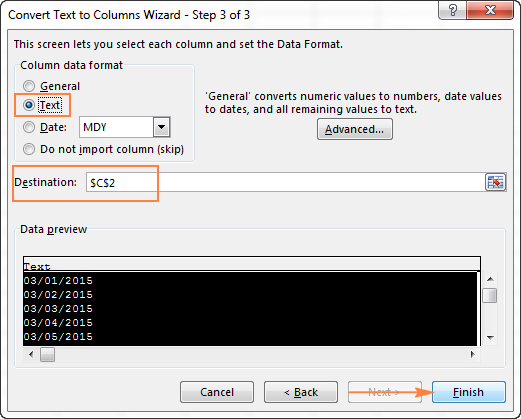That was really easy, right? The screenshot below demonstrates the result - dates converted to text strings in the default short date format set in your Windows Regional settings, which is "mm/dd/yyyy" in my case: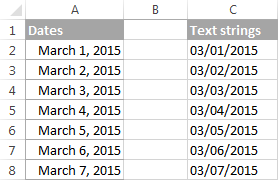## Convert Excel date to text via Notepad

Another quick no-formula way to turn Excel dates into text strings is using Notepad or any other text editor. Unlike the Text to Columns wizard, it allows you to convert Excel date to text in any format of your choosing.

1. In your Excel worksheet, format the dates exactly as you want the text strings to look like.
2. Select all of the dates you want to convert and press Ctrl+C to copy them.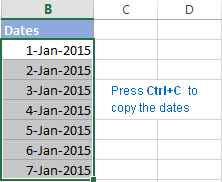3. Open Notepad or any other text editor, and paste the copied dates there.
4. Notepad automatically converts the dates to the text format. Press Ctrl+A to select all text strings, and then Ctrl+C to copy them.
5. Switch back to Microsoft Excel, select the column where you want to insert the text strings and apply the Text format to it. To do this, press Ctrl+1 to open the Format Cells dialog and select Text on the Number tab.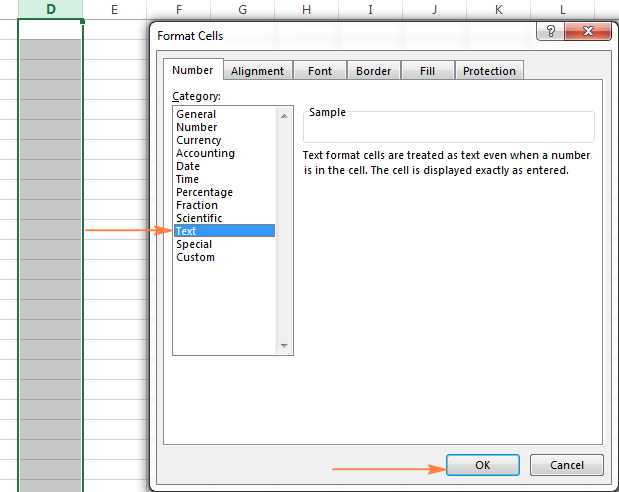6. Finally, select the first cell where you want to insert the text strings and press Ctrl+V to paste them.

The following screenshot shows the result, with the original Excel dates in column B and text entries in column D. Please notice that the converted text strings reflect the original date format with absolute accuracy, except they are left-alighted, as all text values are supposed to be in Excel.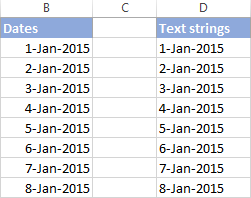This is how you convert date to text in Excel. Next week we will explorer a few Excel functions to work with weekdays and days of the year. And in the meantime, you may want to check out the previous parts of our comprehensive tutorial to working with dates and times in Excel.

## Excel Dates Tutorials:

1. Hi Svetlana,

I am trying to copy a either an entire worksheet or a range of data from one workbook to another. I can do this successfully manually, by selecting all the cells with data in them and pasting into a newly created worksheet (Date format is maintained). However when I try to do this via VBA any date with a day greater than 12 gets converted to text [US (m/dd/yyyy)/Australian (dd/mm/yyyy) format change]. I've tried formating the cells first to US format dates but this doesn't help. Any sugestions?

2. Hi Svetlana.

This blog is awesome. Thanks

I have a column D in an Excel sheet that shows date exported from a system in the format;
day/ month / yyyy as shown in examples below
29/07/2010
24/04/2010
9/7/2010
20/07/2010
15/08/2010
etc

I need to convert these dobs in to a 4 digit PIN code DDMM
so results from above will be:
2907
2404
0907
2007
1508

I've tried =TEXT(D2,"mmdd") but it keeps the slashes / and always retains the yyyy at the end
I've tried =TEXT(D2,"dd")&TEXT(D2,"mm") but same issues

A maths friend gave me this and it seemed to work for a while:
=IF(LEN(TEXT(100*DAY(D2)+MONTH(D2),0))=3,CONCATENATE("0",TEXT(100*DAY(D2)+MONTH(D2),0)),TEXT(100*DAY(D2)+MONTH(D2),0))

I'm using Excel 2010. Help please.

Thank you in anticipation

• Hi Mark,

This simple formula works just fine for me:
=TEXT(D2, "ddmm")

You can check it in a new empty sheet by typing a few dates manually in your default date format, not copy/paste from the existing sheet. If it works with the regular dates typed manually, then most likely the problem is with the exported dates, e.g. they may be text strings that look like dates. To check this, select any cell with a date, and look at the Number Format box on the Home tab > Number group. If it shows Text or General, you are dealing with text strings, not dates. In this case, you can simply extract the 1st, 2nd, 4th, and 5th character using this formula:
=LEFT(D2,2)&MID(D2,4,2)

Please note, the above formula will work only if all the dates are in the same format, i.e. dd/mm/yyyy.

If there are dates formatted as d/m/yyyy (without leading zeros), you will need to convert text strings to dates first, and then apply =TEXT(D2, "ddmm") to the converted dates.

• OK - thanks for confirmation & the extra info

3. that was very simple way of doing.
Is there no other option, using Text and columns??

• Sure, you can use the Text to Columns feature too as demonstrated in this example:
Covert text to date with Text to Columns. I prefer 'Replace All' because it's faster, but it's up to you.

Another way to convert a date in mm.dd.yyyy (or dd.mm.yyyy) format to mm-dd-yyyy (or dd-mm-yyyy) is using the Substitute function:
=VALUE(SUBSTITUTE(A1, ".", "-"))

4. Hi,
I would like to learn, how to convert 01.01.2016 to 01-01-2016 format.

• =SUBSTITUTE(A1,".","-")

5. Hi all,
I would like to convert date, i.e. 31-12-2015 into text format... like Thirty one - December - Two thousand fifteen.

6. I would like to enter a ratio like "1:1" or "7:5" in a cell that has been previously formatted as Text. Excel 2013 constantly changes my entries to date/time formats. How hard is it for the geniuses in Redmond to understand that when I format a cell as Text I want whatever I enter in it to be displayed EXACTLY AS I HAVE ENTERED IT and NOT AS ANYTHING ELSE???????

7. How to use d(), m() and y() functions in excel

8. I have cells formated as text.now i wantto genrate todaydate but in text formate these cells does not read today date.plz help

9. i want to know how to convert date into words(english) in excel 2003 or 2007
for eg. 22.04.2010 - convert it into Twenty Two April 2010 or Twenty Two April Two Thousand Ten.

10. is it possible to covert a numeric date into complete Text format? e.g 13/02/2015 in thirteenth February Two thousand and fifteen.

11. Hi my question is when I am use

=E1&" "&" "&E2&" "&TEXT(E3, "MMM YY ") formula.

I want to change the year from 2016 to 2015 but when copy that formula and past it into the next cell the year stay the same even when I put it as paste fill without formatting.

12. how to convert date for example 14/07/2008 in word " Fourteenth Jun Tow Thousand"

13. i want to know how to convert date into words(english) in excel 2003 or 2007
for eg. 22.04.2010 - convert it into Twenty Two April 2010 or Twenty Two April Two Thousand Ten.

14. 27/5/2016
21/4/2016
29/5/2016
15/5/2016

not able to change this date format to mm/dd/yy

• Hello Priti,

Select the cells, pres Ctrl+1 to open the Format Cells dialog, select Date under Category and choose the desired format. Or, select Custom under Category, and type mm/dd/yy in the Type box.

16. how to convert to date month year words in excel

17. Hi Mam! Could you help me convert "Sat, May 14, 2016" into "14/5/2016" format and separate "Sat" from the string when the former started with asterisk mark. Thanks in advance.

19. Hi Svetlana
how to convert date of birth in text
02/02/1966- Second February nineteen sixty six in Excel sheet
pl what formula i have to write
my mob.no is 7773876579

20. how to convert date of birth in text
01/07/1965- First july nineteen sixty five in Excel sheet
pl what formula i have to write.my mob.number is 7773876579

21. Hi Svetlana,

When I use the Text function within a formula, it is still displaying a number. Any reason why it is doing it? Note that when I try the function in a standalone cell, its working perfectly fine.

Here's what I mean-

="select ric_code,exchange_code,expiry_date from smd_live_raw..t_ds_optfuture where expiry_date =TEXT(42587,"dd-mmm-yyyy")"

The number 42587 is being taken from a cell that contains a date.

-Kishore

• Please change general function after use this formula TEXT(42587,"DD MMM YY")
ans : 05 Aug 16

22. how do u create if formula for
if 06:00:00 to 9:00:00 should be P
after 11:00:00 AM should be HAL
Before 2 PM should be HAL again

23. Hi there,

Please help me - I have this birth date that I want to change to text ie 19720101 should read 01 Jan 1972, how do I do that?

Thank you so much!
Thersia

• Hello THERSIA,

From your example, it's not quite clear which of the two "01" in 19720101 is month and which is day. Assuming it is the "yyyymmdd" format, you can use one of the following formulas (where A1 contains 19720101):

To convert the number to a date:

=DATE(LEFT(A1,4),MID(A1,5,2),RIGHT(A1,2))

As the result, the formula returns a serial number representing the date. Now, select the formula cell, press Ctrl+1 and set the following date format: "dd mmm yyyy". Please see How to create a custom date format in Excel for the detailed steps.

To convert the number to a text string:

=TEXT((DATE(LEFT(A1,4),MID(A1,5,2),RIGHT(A1,2))), "dd mmm yyyy")

24. When I paste the column to notepad - it does not change it to text. When I paste it back in excel - it is still a date. I am using office 365

25. Hi
I used some software for entry so I export my entries and worked for invoice date + 30 = schedule date
In our report 1 to 12 date its calculated but 13 to 31 dates calculation its not able and the answer its #value

26. How to convert Mon, 30 Nov, 2015 to 30-Nov-2015 format

27. I have the same question as above.

date format example 01/01/2015 say in cell no a1
i want to convert as follows 01012015 in cell no b1

But when I tried what you recommended it didn't work.

=TEXT(A1,"mmddyyyy")
=TEXT(A1,"ddmmyyyy")

Is there another way? Thank you so much for the help!

• Hi Tiffany,

I've just tried the formula on my sheet and it has worked fine. How exactly doesn't it work in your Excel? Does it return a wrong result or error? If the latter, what error?

28. Hi Svetlana,

I hope you can help me. I have 9/1/2015 12:11:53 PM (cell A2) which i need to convert into text. I have used some of the idea above which is =text(A2,"dd/mm/yyyy hh;mm;ss"), some cell works but some cell doesn't.
Do you know is there any other ways to convert this? Thanks in advance.

• Hi Yennie,

In the time part of your format, try replacing semicolons with colons, like this:

=TEXT(A2,"dd/mm/yyyy hh:mm:ss")

29. Ah - just saw your post; many thanks!

30. Cracked it!

=IF(ISBLANK(N2);"";(TEXT(N2;"mmm-yy")))

31. Hi Svetlana,

Thanks for such an informative post.

Is there a way to construct a formula like the below to return nothing if the source cell is blank? Right now, it is returning Jan-OO for all empty cells.

=TEXT(N2;"mmm-yy")

Thanks,
Donal

• Hi Donal,

Sure. You can use the IF function to check for blank cells, like this:
=IF(N2=""; ""; TEXT(N2;"mmm-yy"))

32. Hi Svetlana,

Wondering if you could help me. I have a date in cell AB5, in US english format (mm/dd/yyyy) and a formula =TEXT(AB5, "mmm/yy") in cell AX5. For some reason the formula returns the date in date format rather than my desired Mon/YY format- any ideas why this may be the case?

Best regards

Jon

33. Hi i i have 14/10/2015 11:01
14/10/2015 11:01
14/10/2015 11:01
14/10/2015 11:01
14/10/2015 11:02
14/10/2015 11:02
14/10/2015 11:02
14/10/2015 11:02
14/10/2015 11:02
14/10/2015 11:02
14/10/2015 11:02
14/10/2015 11:02

i want to add am/pm to each one. the date is currently in text mode.

34. Hi
I want formula For text Date in Excel like that
Date:20/10/2015
"Twenty ,Ten, Two Thousand Fifteen"

35. good evening Sir,
how to convert date of birth in text
02/02/1966- Second February nineteen sixty six in Excel sheet
pl what formula i have to write

36. Is there a way to convert a birthday (01/15/2012) into number of months and days (45 months 1 day)? I need the number of months and days to keep a continuous count as of the current day.
Thanks.

• Hi Patty,

You can use the following formula, where B2 is the cell containing a birthday date:

=DATEDIF(B2,TODAY(),"M") & " Months " & DATEDIF(B2,TODAY(),"MD") & " Days"

37. Is there a way to sort the text dates in chronological order in a pivot once you've used the formula in the data?

I'm doing a rolling year oct-2014 through sept-2015 (displayed as 15-Sep with text formula) but when pivoting this the dates show as alphabetized (ie 14-dec,14-oct,14-nov,15-apr,15-aug and so on)

38. I WANT TO LEARN DATE CONVERT INTO WORDS SUCH AS 01/01/2005,ONE JANUARY TWO THOUSAND FIVE

39. Hello, i just want to know the formula if i input "date" from cell1, and on cell2, output will be the year only. Like for example if i input date 9/29/2015 on cell1, the output for cell2 must be "A" for year 2015. Then if year 2016, the output will be B, and so on. Thanks.

40. Good news... I figured it out. This is how I did it:
For B5, function is =IF(OR(\$K\$2="",\$L\$2=""),"",TEXT(EDATE(\$K\$2,1),"mmm 'yy"))
For C5, function is =IF(OR(\$K\$2="",\$L\$2=""),"",TEXT(EDATE(\$K\$2,2),"mmm 'yy"))

and so on.

41. Hi Svetlana,

Do you know of a way to increment months from a date value input so it'll automatically show up in correct month/year order in a table?

Example: I have 12 columns, A to L. In K2, I input 9/20/15 (as the Start Date).
Then for A5 to L5, I retrieve from K2 and it should automatically increment month/year (as needed).
A5 has formula of =IF(OR(\$K\$2="",\$L\$2=""),"",TEXT(\$K\$2,"mmm 'yy"))
The output for A5 is Sept '15
I want B5 to automatically become Oct '15, C5 to become Nov '15, D5 to become Dec '15, E5 to become Jan '16, and so on.
Any suggestions on how to do this?
Many thanks.

42. Hi,

Is there a formula to convert 18/09/2015 to "Weekday" or 6/09/2015 to "Weekend"?

Thanks,

• Hi JP,

You can use the following formula:
=IF(WEEKDAY(A1, 2)<6, "Weekday", "Weekend")

Where A1 is the cell with a date.

43. sir,my birthday 06-04-1983 ko excel formula se convert word six april nineteen eghty three

• Sir,Can you help me in converting date into wordssuch as 05.04.1995 into Fifth, April, Nineteen Ninety five

I want to mark "P" if the time-value is <7:00:00 hrs

• Hello Azhar,

Supposing your have a list of times in column A, you can use the following formula:
=IF(A1<TIMEVALUE("7:00:00"), "P", "")

• how to convert date of birth in text 02/02/1966- Second February nineteen sixty six in Excel sheet pl what formula i have to write
Plese help me..

• Sunil,

I don't know a formula that could do this. Most likely a macro is needed in this case.

45. Hi!

I tried so much to find a way that i could use the number of the week of the year and the day of the week and make it a number. For example if we are in the 34th week of the year and the day is Tuesday then the text should show 3402. If its the 24th week of the year and the day is Monday then the text should show 2301 . The first 2 digits are the week of the year, and the other 2 digits are the monday-tuesday etc. Could you pls help me?

• I did it :) see you

46. Hi:

Thanks to you awesome tutorial I was able to convert date to text, but know I want to convert that text value into something measurable MINUTES.

For example:
My (A2) cell value is 1d:03h:40m  1day + 3hours + 40 minutes  (1*24*60)+(3*60)+40 =1660

Can you please advise how can I perform or execute this conversion from TEXT(Date) to NUMBER (Minutes)?

Any questions feel free to let me know.

• Hi!

1d:03h:40m is a very specific format, so the formula is also highly specific:

=VALUE(LEFT(A2,FIND("d",A2)-1))*24*70+VALUE(MID(A2,FIND("h",A2)-2,2))*70+VALUE(MID(A2,LEN(A2)-2,2))

Please note that it will work correctly only if hours and minutes always contain 2 digits, with leading zeros if needed.

47. Hi;

I want to convert number of days to y m d.
Eg; 1092 days and have Excel convert that to 2years 11months 6days

Thankfull for quick replay.

• Hi!

Because there are leap and non-leap years, and the number of days in each month varies from 28 to 31, we can only suggest the formula with a certain degree of approximation. I.e. 365 days are counted as a year, and 365/12 is counted as a month:

=CONCATENATE(IF(INT(A1/365)>0,INT(A1/365)&" years",""),IF(INT(MOD(A1,365)/(365/12))>0," "&INT(MOD(A1,365)/(365/12))&" months", "")," ",IF((A1-INT(A1/365)*365-INT(MOD(A1,365)/(365/12))*(365/12))>0,INT((A1-INT(A1/365)*365-INT(MOD(A1,365)/(365/12))*(365/12)))&" days",""))

Where A1 is the cell containing the number of days you want to convert.

For 1092 days, the formula returns 2 years 11 months 27 days.

• Thanks so much it working :)

• i want some more i dont have days in there i have only Date of Joing a one person then i want everything do in a one cell.

• Syed,

If you want to display the difference between 2 dates, say between the Date of Joining (A1) and today's date, then you can use the DATEDIF function to calculate the date difference, and the formula is much simpler:

=DATEDIF(A1, TODAY(), "y") &" years, "&DATEDIF(A1, TODAY(), "ym") &" months, " &DATEDIF(A1, TODAY(), "md") &" days"

48. Hello,

Please help me some time if i save the Excel totally text format is changing to Date format.

Now i need to change total Excel format Date format to Text format how can i change the format.

date format example 01/01/2015 say in cell no a1
i want to convert as follows 01012015 in cell no b1

• Hi HABIBUL,

You can enter one of the following formulas in B1, depending on which time unit you want to come first - month or day:

=TEXT(A1,"mmddyyyy")
=TEXT(A1,"ddmmyyyy")

• Dear Svetlana, I am using Excel 2010 v 14.0.473
When using the formula =text(A1,"ddd") I get "The formula you typed contains an error". A1 = 2016/11/17

• Hello Riaan,

It may happen if a value in A1 is a text string, not a date. To check this, select A1 and look at the Number Format box on the Home tab in the Number group. If it displays General or Text, you are dealing with a text string that looks like a date. In this case, you will need to convert text to date first.

• Dear Svetlana, nope, the format is Date. Even if I point the formula to an empty cell, I get the same error. This does not seem right.

Even when I use Datevalue and point to my date field that is either formatted as text or date, I get #VALUE!

All other Excel formulas work perfectly.

Unfortunately I cannot post screen shots here of my spread sheets to prove my points to you.

Any suggestions?

• Dear Riaan,

I am afraid it's not possible to determine the root of the problem without seeing your data. If you can send us your sample worksheet at support@ablebits.com, I will try to help.

50. Hai,